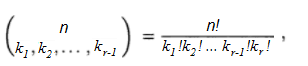# Multinomial Coefficient: Simple Definition

Share on

The multinomial coefficient, used in combinatorics, is an extension of the binomial coefficient. It’s used to find permutations when you have repeating values or duplicate items. The formula is:In formal terms, the multinomial coefficient formula gives the expansion of (k1 + k2 … + kn) where ki are non-negative integers. Informally, you can think of it as a way to find how many permutations are possible when you have duplicate values for k. This is best illustrated with an example.

## Multinomial Coefficient Example

A practical example of when you might use the multinomial coefficient is given by Harris et. al in Combinatorics and Graph Theory . Let’s say you wanted to count the number of ways to order n objects. If the objects are all different, then there are n! ways to order them. But if some of the objects are a multiset and some of the objects are the same, n! will produce too many permutations.

For example, let’s say you’re trying to find the number of different permutations for the letters in the word Mississippi. Note there are actually only four unique letters: MISP. The number of times the letters appear:

• M = 1
• I = 4
• S = 4
• P = 2

Inserting those values into the multinomial coefficient formula, where n is the total number of letters in the word MISSISSIPPI, and kn is the individual letter count (from the above list):
n! / k1! * k2! * k3! * k4! = 11! / ( 1! * 4! * 4! * 2! ) = 11! / ( 1 * 24 * 24 * 2 ) = 34,650.

Note that n! gives 11! = 39916800, which is way larger than the actual number of distinguishable permutations.

You can think of the multinomial coefficient then, as a “permutations correction” when there are duplicate items in the counting set.

References:
John Harris, Jeffry L. Hirst, Michael Mossinghoff. Combinatorics and Graph Theory.
.

CITE THIS AS:
Stephanie Glen. "Multinomial Coefficient: Simple Definition" From StatisticsHowTo.com: Elementary Statistics for the rest of us! https://www.statisticshowto.com/multinomial-coefficient/
---------------------------------------------------------------------------Need help with a homework or test question? With Chegg Study, you can get step-by-step solutions to your questions from an expert in the field. Your first 30 minutes with a Chegg tutor is free!